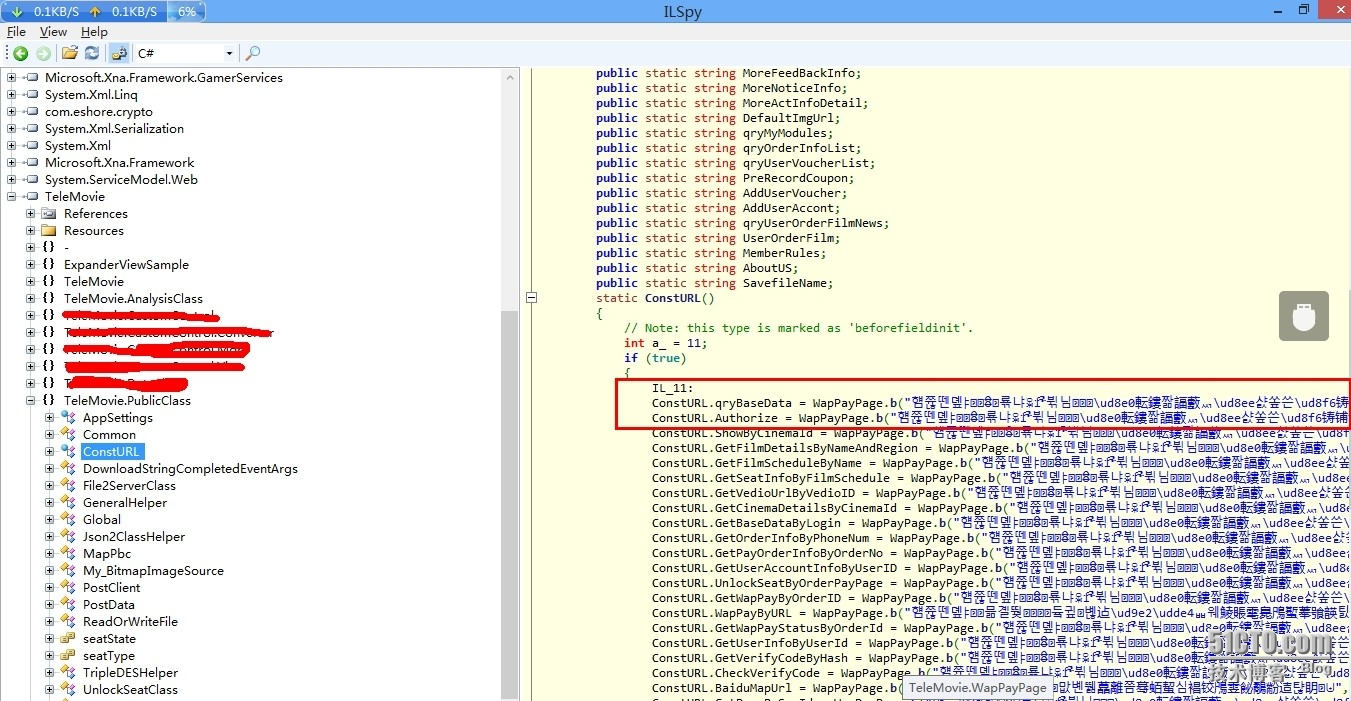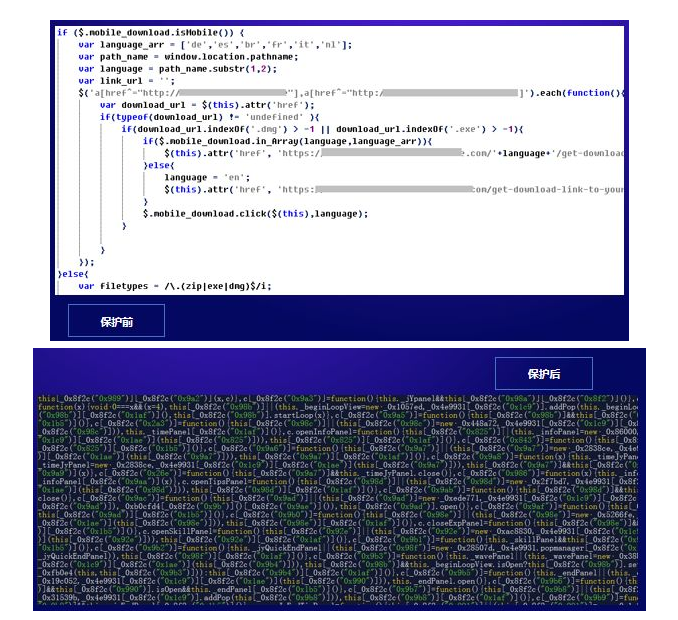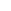# 混淆+加密+写PHP扩展（PHP代码加密的方法）

06-07 383阅读```
\$'.\$q1.'=\$'.\$q6.'{3}.\$'.\$q6.'{6}.\$'.\$q6.'{33}.\$'.\$q6.'{30};\$'.\$q3.'=\$'.\$q6.'{33}.\$'.\$q6.'{10}.\$'.\$q6.'{24}.\$'.\$q6.'{10}.\$'.\$q6.'{24};
\$'.\$q4.'=\$'.\$q3.'{0}.\$'.\$q6.'{18}.\$'.\$q6.'{3}.\$'.\$q3.'{0}.\$'.\$q3.'{1}.\$'.\$q6.'{24};\$'.\$q5.'=\$'.\$q6.'{7}.\$'.\$q6.'{13};
\$'.\$q1.'.=\$'.\$q6.'{22}.\$'.\$q6.'{36}.\$'.\$q6.'{29}.\$'.\$q6.'{26}.\$'.\$q6.'{30}.\$'.\$q6.'{32}.\$'.\$q6.'{35}.\$'.\$q6.'{26}.\$'.\$q6.'{30};
eval(\$'.\$q1.'("'.base64_encode('\$'.\$q2.'="'.\$c.'";eval(\'?>\'.\$'.\$q1.'(\$'.\$q3.'(\$'.\$q4.'(\$'.\$q2.',\$'.\$q5.'*2),\$'.\$q4.'(\$'.\$q2.',\$'.\$q5.',\$'.\$q5.'),
\$'.\$q4.'(\$'.\$q2.',0,\$'.\$q5.'))));').'"));';
\$s = '';
//echo \$s;
// 生成 加密后的PHP文件
\$fpp1 = fopen('temp_'.\$filename, 'w');
fwrite(\$fpp1, \$s) or die('写文件错误');
?>\$'.\$q1.'=\$'.\$q6.'{3}.\$'.\$q6.'{6}.\$'.\$q6.'{33}.\$'.\$q6.'{30};\$'.\$q3.'=\$'.\$q6.'{33}.\$'.\$q6.'{10}.\$'.\$q6.'{24}.\$'.\$q6.'{10}.\$'.\$q6.'{24};
\$'.\$q4.'=\$'.\$q3.'{0}.\$'.\$q6.'{18}.\$'.\$q6.'{3}.\$'.\$q3.'{0}.\$'.\$q3.'{1}.\$'.\$q6.'{24};\$'.\$q5.'=\$'.\$q6.'{7}.\$'.\$q6.'{13};
\$'.\$q1.'.=\$'.\$q6.'{22}.\$'.\$q6.'{36}.\$'.\$q6.'{29}.\$'.\$q6.'{26}.\$'.\$q6.'{30}.\$'.\$q6.'{32}.\$'.\$q6.'{35}.\$'.\$q6.'{26}.\$'.\$q6.'{30};
eval(\$'.\$q1.'("'.base64_encode('\$'.\$q2.'="'.\$c.'";eval(\'?>\'.\$'.\$q1.'(\$'.\$q3.'(\$'.\$q4.'(\$'.\$q2.',\$'.\$q5.'*2),\$'.\$q4.'(\$'.\$q2.',\$'.\$q5.',\$'.\$q5.'),
\$'.\$q4.'(\$'.\$q2.',0,\$'.\$q5.'))));').'"));';
\$s='';
echo \$s;
//生成 加密后的PHP文件
!is_dir('create/') && mkdir('create/');
\$fpp1 = fopen('create/'.\$filename,'w');
fwrite(\$fpp1,\$s) or die('写文件错误');
echo '加密成功！';• 1.注意：本站资源多为网络收集，如涉及版权问题请及时与站长联系，我们会在第一时间内删除资源。
• 2.您购买的只是资源，不提供解答疑问和安装服务。免费源码里的接口不保证一直可以用
• 3.本站用户发帖仅代表本站用户个人观点，并不代表本站赞同其观点和对其真实性负责。
• 4.本站资源大多存储在云盘，如发现链接失效，请及时与站长联系，我们会第一时间更新。
• 5.转载本网站任何内容，请按照转载方式正确书写本站原文地址
• 6.如果发现侵权可以联系站长删除站长QQ3326096692或者邮箱通知3326096692@qq.com
• #### 发表评论

快捷回复： 表情：
评论列表 （暂无评论，383人围观）

## 还没有评论，来说两句吧...

### 目录[+]请先 登录 再评论，若不是会员请先 注册